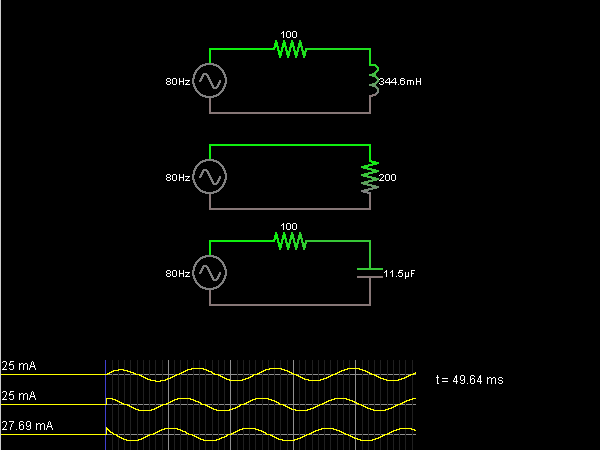# Circuit Simulator - Impedances of Same Magnitude

Impedances of Same MagnitudeCircuit Description:
These three circuits have the same AC source driving three different impedances. The impedance has the same magnitude in each case, but different phases. In the middle case, the impedance is purely resistive and has no reactance. In the other two cases (with an inductor and a capacitor) there is some reactance present, so the phase of the current is different. In all three cases, the peak value of the current is the same (25 mA).

Discussion:
Be the first person to comment on this question !